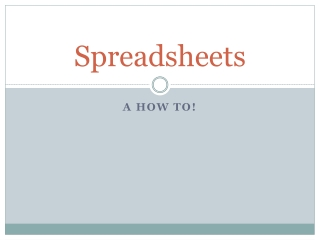# Spreadsheets - PowerPoint PPT PresentationDownload PresentationDownload Presentation- - - - - - - - - - - - - - - - - - - - - - - - - - - E N D - - - - - - - - - - - - - - - - - - - - - - - - - - -
##### Presentation Transcript

2. Identifying Labels and Values labels values

3. Identifying Formulas and Functions formula The result of the formula function The result of the function

4. Formula or Function? • =C2+D2+E2 • =AVERAGE(C2:M2) • =E2/B2 • =IF(3A>2A, ‘True’, ‘False’) • =SUM(E4:G4) • =(A2+B2+C2)/3

5. Identifying Parts of a Spreadsheet Formula mathematical operators =B2+C2+D2 equal sign—first part of any spreadsheet formula cell reference/address—made up of the column heading and the row number

6. Identifying Parts of a Spreadsheet Function =SUM(B2:B6) name of function range equal sign

7. What is the active cell?

8. Simple Calculations - Formula

9. Simple Calculations - Formula

10. Simple Calculations - Formula

11. Simple Calculations - Formula

12. Simple Calculations - Formula

13. Simple Calculations - Formula

14. Simple Calculations - Formula

15. Simple Calculations - Formula

16. Simple Calculations – Format Number

17. Simple Calculations – Format Number

18. Simple Calculations - Function

19. Simple Calculations - Function

20. Simple Calculations - Function

21. Simple Calculations - Function

22. Simple Calculations - Function

23. Simple Calculations - Function

24. Simple Calculations - Function

25. How do you calculate this?

26. How do you calculate this?

27. Drag and Drop

28. Drag and Drop

29. Drag and Drop

30. Relative and Absolute Referencing • Relative • cells that change when they are copied into other cells • Absolute • cells that do not change regardless of where it is copied

31. Absolute Cell Reference – F4

32. Drag and Drop